• 值传递和地址传递

值传递和地址传递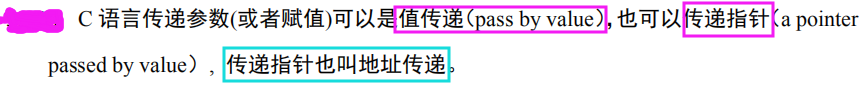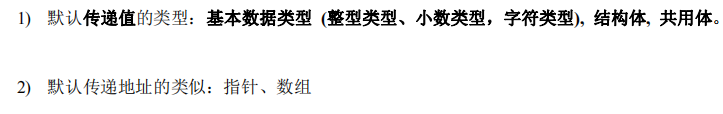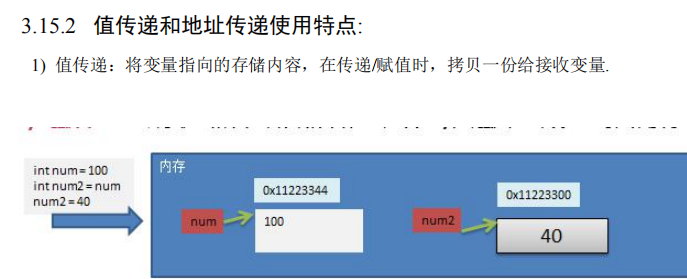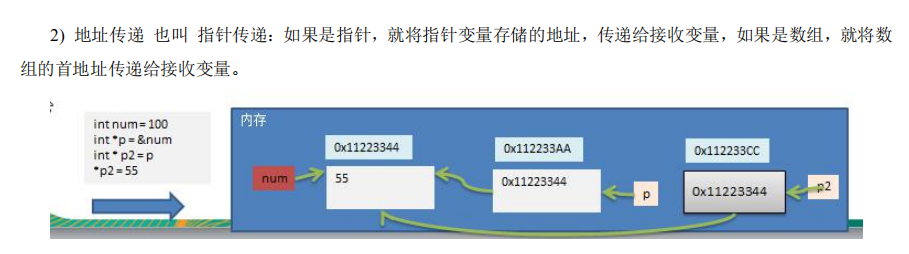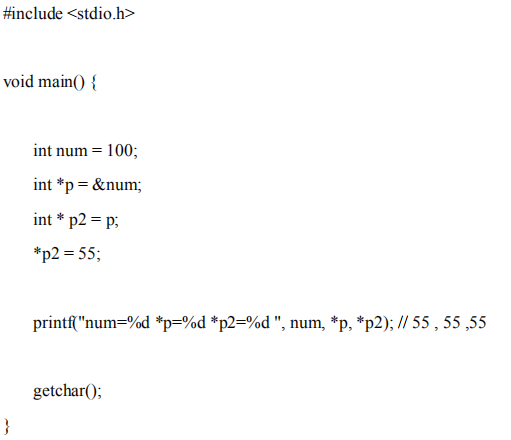展开全文• C++ 值传递和地址传递 在我们在函数间数据传递的时候会出现，在函数中数据处理的间而过理想的，但在主函数中数据并没有发生变化。具体详情如下： 在数据传递时，分为值传递和地址传递； 值传递：在函数调用时实参将...
C++ 值传递和地址传递和引用传递
在我们在函数间数据传递的时候会出现，在函数中数据处理的间而过理想的，但在主函数中数据并没有发生变化。具体详情如下：
在数据传递时，分为值传递和地址传递；
值传递：在函数调用时实参将数据传入形参
#include<iostream>
#include<string>
using namespace std;
void swap(int x, int y)
{
int temp=0;
temp = x;
x = y;
y = temp;
cout << "x=" << x << "   " << "y=" << y << endl;
}
int main()
{

int x = 5;
int y = 6;
cout <<"原始数值为："<< "x=" << x << "   " << "y=" << y << endl;
swap(x,y);
cout <<"在main函数中使用swap函数后的数值为："<< "x="<<x<<"   "<<"y="<<y << endl;
return 0;
}

运行结果为：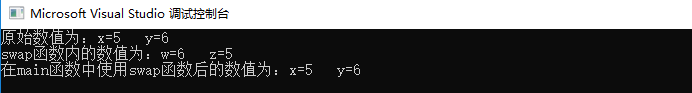结果显示，在swap函数中数据显示确进行了交换，但是在main函数中调用函数后显示，两个数据依然是和原始数据一样。
这是为什么呢？
因为在x,y 给swap传递参数的时候仅仅把数值传递给了形参，函数内的数据的交换，对函数意外的数据结果不起任何的影响，
即：在进行值传递时，函数的形参数据的变化不会改变实参的数据。
那么如何才能让函数中的形参的修改的同时，实参也发生变化呢？这时候就需要用到地址传递或者引用传递。
地址传递：将实参的地址传递给函数的形参
#include<iostream>
using namespace std;

void swap(int * p1, int * p2)
{
int temp;
temp = *p1;
*p1 = *p2;
*p2 = temp;
}
int main()
{
int a = 10;
int b = 20;
swap(&a,&b);
cout <<"a=" << a << "   " << "b=" << b << endl;

return 0;
}

结果为：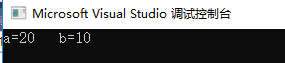此时结果显示，在main中的实参确实发生了变化，这是因为函数的参数为两个指针，此时传递进去的已经不是数据了而是两个数据的内存地址，是在地址上进行的数据的交换，既然地址上的数据发生了变化，两个地址又分别对应实参a和b，即a和b的数据发生了变化。
引用传递：利用引用用形参修饰实参
形式：数据类型 &变量名 = 变量名
例如：
int a =10;
int &b = a;//这是变量a被b引用，即a和b指向同一块内存

参数传递程序：
#include<iostream>
#include<string>
using namespace std;

void swap(int &x,int &y)
{
int temp;
temp = x;
x = y;
y = temp;

}

int main()
{
int a = 10;
int b = 20;

swap(a,b);

cout << "a=" << a << endl;
cout << "b=" << b << endl;

return 0;
}

结果：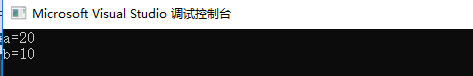结果显示，用引用传递可以用形参修饰实参
引用注意事项：
引用必须初始化
引用在初始化后不可再改变
引用
引用的本质就是在C++内部实现一个指针常量，
例如：
int &ref = a;   编译器内部会自动转化为：int * const ref = &a;    这也解释了为什么引用一旦初始化不能再改变。
常量引用：
作用：const 修饰引用后，引用的数值不可再修改
注意：
int & ref = 10;//这样写是错误的，因为引用必须是一个合法的地址
const int & ref = 10;//这样写是正确的，加上const后，编译器将代码修改为 int temp = 10; int & ref = temp;



展开全文• 值传递和地址传递图解
值传递和地址传递图解
class Test3 {
public static void main(String[] args) {
int a = 10;
int b = 20;
System.out.println("a:" + a + ",b:" + b);
change(a, b);
System.out.println("a:" + a + ",b:" + b);
//a=10,b=20
//a=10,b=20
//a=20,b=40
//a=10,b=20  值传递
int[] arr = new int[]{ 1, 2, 3, 4, 5 };
change(arr);
System.out.println(arr);//4
System.out.println(arr);//8

}

public static void change(int a, int b) {
System.out.println("a:" + a + ",b:" + b);
a = b;
b = a + b;
System.out.println("a:" + a + ",b:" + b);
}

public static void change(int[] arr) {

//arr=new int[]{1,2,3,4,5};

for (int x = 0; x < arr.length; x++) {
if (arr[x] % 2 == 0) {
arr[x] *= 2;
}
}
}
}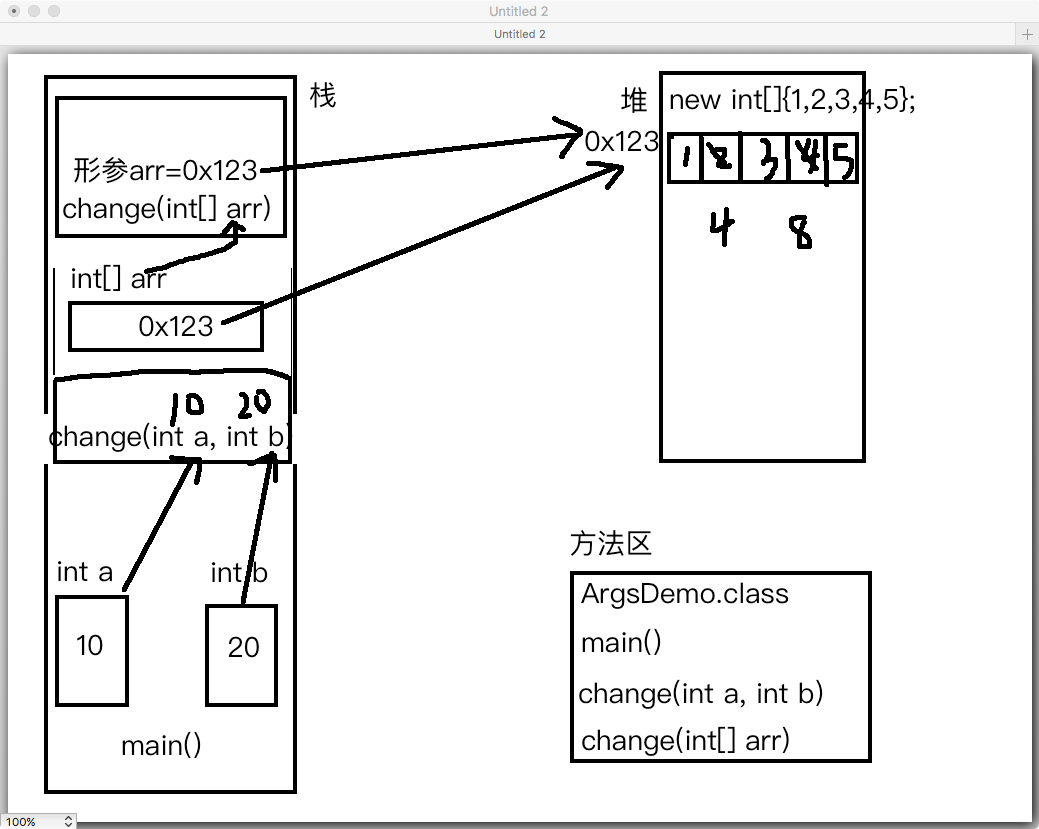展开全文java知识
• 值传递和地址传递用到了栈与堆的知识。 我们来看一部分代码示例； 从图中我们可以看出值传递是不会改变a的值，虽然将a的值赋值给了b，但是b的修改不会影响a的内容。 对于地址传递，假设有数组array与ages，将array...
值传递和地址传递用到了栈与堆的知识。
我们来看一部分代码示例；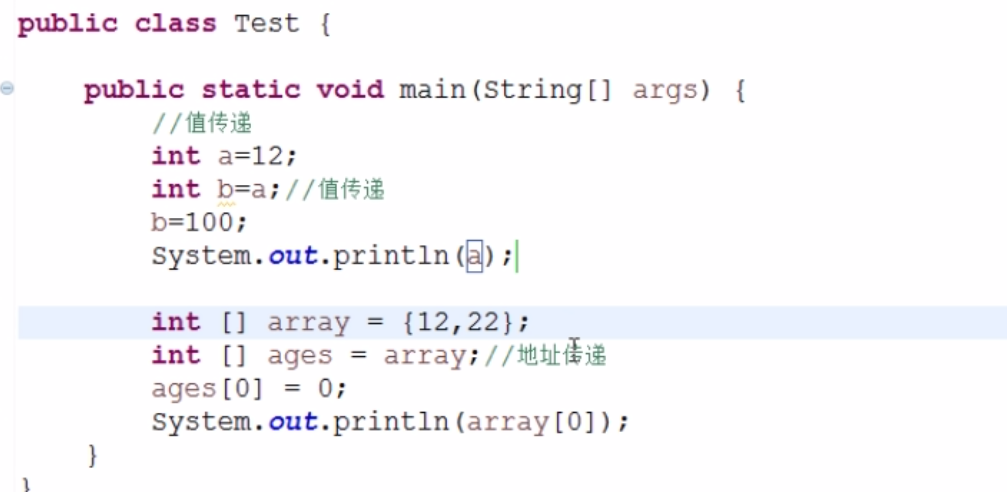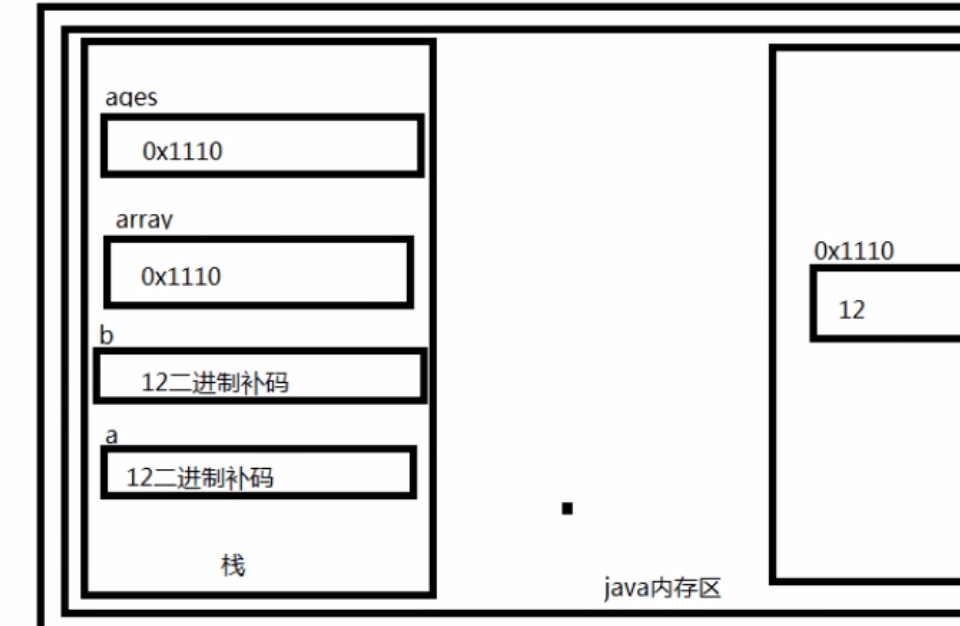从图中我们可以看出值传递是不会改变a的值，虽然将a的值赋值给了b，但是b的修改不会影响a的内容。
对于地址传递，假设有数组array与ages，将array数组赋值给ages数组，其实是将array的地址赋值给了ages数组，在栈中，他们指向同一地址，所以一旦两者中任何一个数组的内容发生改变，数组的内容就会改变。


展开全文java
• 值传递和地址传递，C语言函数传参方式详解 C语言中文网推出辅导班啦，包括「C语言辅导班、C++辅导班、算法/数据结构辅导班」，全部都是一对一教学：一对一辅导 + 一对一答疑 + 布置作业 + 项目实践 + 永久学习。QQ...
• java学习-值传递和地址传递 java的面向对象中学到了值传递和地址传递，由于这个知识多次搞错，在此记录一下。 值传递和地址传递： 如果变量是基本数据类型，此时赋值的是变量所保存的数据值。 如果变量是引用数据...
• 值传递和地址传递 C/C++里面声明一个函数的同时可以传入一些参数，称之为形式参数（形参），在传输参数时，有值传递和地址传递，如： #include<stdio.h> void fun(int c); //值传递 void fun_p(int *d); //......